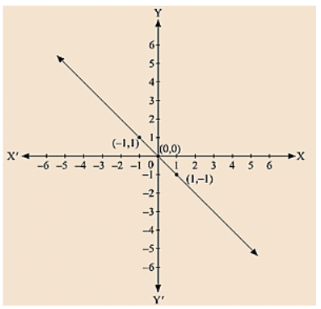# From the choices given below, choose the equations whose graph is given in fig

Question:

From the choices given below, choose the equations whose graph is given in fig

(i) y = x

(ii) x + y = 0

(iii) y = 2x

(iv) 2 + 3y = 7x

Solution:

We are given co-ordinates (1, - 1) and (-1, 1) as the solution of one of the following equations.

We will substitute the value of both co-ordinates in each of the equation and find the equation which satisfies the given co-ordinates.(i) We are given, y = x

Substituting x =I and y = - 1, we get; 1 ≠ -1

L.H.S ≠ R.H.S

Substituting x = -1 and y = 1, we get; – 1 ≠1

L.H.S ≠ R.H.S

Therefore, the given equation y = x does not represent the graph in the figure.

(ii) We are given, x + y = 0

Substituting x = 1 and y = -1, we get

⟹ 1 + (-1) = 0

⟹ 0 = 0

L.H.S = R.H.S

Substituting x = 1 and y = 1, we get (-1) + 1 = 0

0 = 0

L.H.S = R.H.S

Therefore, the given solutions satisfy this equation.

Thus, it is the equation whose graph is given.